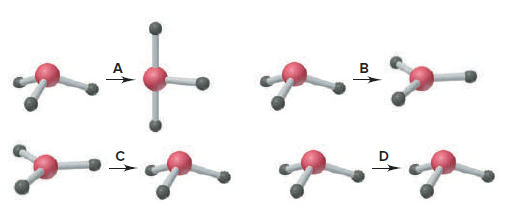# Problem: When SO3 gains two electrons, SO32− forms. (a) Which depiction best illustrates the change in molecular shape around S?

###### FREE Expert Solution

Concept: Molecular Geometry

Let's first draw the Lewis Dot Structure of SO3 to determine it's molecular geometry.

95% (357 ratings)###### Problem Details

When SO3 gains two electrons, SO32− forms. (a) Which depiction best illustrates the change in molecular shape around S?Frequently Asked Questions

What scientific concept do you need to know in order to solve this problem?

Our tutors have indicated that to solve this problem you will need to apply the Electron Geometry concept. You can view video lessons to learn Electron Geometry. Or if you need more Electron Geometry practice, you can also practice Electron Geometry practice problems.

What professor is this problem relevant for?

Based on our data, we think this problem is relevant for Professor Maxwell's class at UCF.

What textbook is this problem found in?

Our data indicates that this problem or a close variation was asked in Chemistry: The Molecular Nature of Matter and Change - Silberberg 8th Edition. You can also practice Chemistry: The Molecular Nature of Matter and Change - Silberberg 8th Edition practice problems.